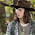## Saturday, August 5, 2017

Dynamics of structures is a special branch of structural analysis, which deals with the behaviour of structures subjected to dynamic loads (loads that vary with time). Such loads develop dynamic reactions, internal forces, and  displacements in the structure. These values all change with time, and maximum values often exceed static load reaction values. Dynamic analysis of any structure often begins with the free vibration analysis.

A structure undergoes free vibration when it is brought out of static equilibrium and can then oscillate without any external dynamic excitation. Free vibration of structures occurs with some frequencies which depend only on the parameters of the structures such as boundary conditions, distribution of masses, stiffnesses within the members etc, and not on the reason for the vibration. At each natural frequency of free vibration, the structure vibrates in simple harmonic motion where the displaced shape (mode shape) of the structure is constant but the amplitude of the displacement is varying in a sinusoidal manner with time. The number of natural frequencies in a structure coincides with the number of degrees of freedom in the structure. These frequencies are inherent to the given structure and are often referred to as eigenfrequencies. Each mode shape of vibration shows the form of an elastic curve which corresponds to a specific frequency.

A truss is loaded as shown below with a lumped mass of 5000kg. We are to obtain the eigenfrequencies and mode shapes by assuming linear behaviour, neglecting damping, and assuming that the stiffness and inertial effects are time independent.

E = 205 KN/mm2
A = 0.00548 m2 (UB 254 x 146 x 43)

A little consideration will show that the structure has two degrees of freedom which are given by the vertical and horizontal translation at node C. The lumped mass at the node will definitely participate in those movements. The displacements are given by Y1 and Y2 below.

Geometrical Properties
θ = tan-1(3/4) = 36.869°
cos θ = 0.8
sin θ = 0.6
LAC = √(32 + 42) = 5m

γ = tan-1(4/4) = 45°
cos γ = 0.7071
sin γ = 0.7071
LBC = √(32 + 32) = 4.243m

ANALYSIS OF STATE 1; Y1 = 1.0

∑Fy = 0
-0.6FAC - 0.7071FCB = 1.0 ----------- (1)

∑Fx = 0
-0.8FAC + 0.7071FCB = 0 ----------- (2)

Solving (1) and (2) simultaneously
FAC = -0.7143
FCB = -0.8081

ANALYSIS OF STATE 2; Y2 = 1.0
∑Fy = 0
-0.6FAC - 0.7071FCB = 0 ----------- (3)

∑Fx = 0
-0.8FAC + 0.7071FCB = -1.0 ----------- (4)

Solving (3) and (4) simultaneously
FAC = 0.7143
FCB = -0.6061

The frequency equation of a 2 degree of freedom system in terms of displacement is given by;

Where;
M is the lumped mass
A is the amplitudes (Note that the system does not allow us to find the amplitudes but we can find the ratio of the amplitudes)
ω is the angular velocity
And of course δij is the axial displacement given by;

For the system above, we can easily compute this;

δ11 = 1/EA[(-0.7143 × -0.7143 × 5) + (-0.8081 × -0.8081 × 4.243)] = 5.3219/EA

δ22 = 1/EA[(0.7143 × 0.7143 × 5) + (-0.6061 × -0.6061 × 4.243)] = 4.1098/EA

δ21 = δ12 = 1/EA[(-0.7143 × 0.7143 × 5) + (-0.6011 × -0.8081 × 4.243)] = -0.4729/EA

To simplify our calculations, let δ0 = 1/EA
Also let λ = 1/Mδ0ω2

Therefore;

(5.3219 - λ)A1 - 0.4729A2 = 0
0.4729A1 + (4.1098 - λ)A2 = 0

On expanding;
λ2 - 9.4317λ + 21.6484 = 0

λ1 = 5.4845
λ2 = 3.9472

You can verify that ω2 = EA/λM

Therefore;
ω12 = (0.1823 × 205 × 106 × 0.00548)/5000 = 40.959
ω22 = (0.2533 × 205 × 106 × 0.00548)/5000 = 56.911

Hence;

To obtain the mode shapes;

For the first mode of vibration; Let A1 = 1.0
(5.3219 - λ)A1 - 0.4729A2 = 0
(5.3219 - 5.4845) - 0.4729 A2 = 0
Therefore, A2 = -0.1626/0.4729 = -0.344
Φ1 = [1.000 -0.344]T

For the second mode of vibration; Let A1 = 1.0
(5.3219 - λ)A1 - 0.4729A2 = 0
(5.3219 - 3.9472) - 0.4729 A2 = 0
Therefore, A2 = 1.3737/0.4729 = 2.9069
Φ2 = [1.000 2.907]T

The modal matrix is then defined as;

Remember Structville....

We have an interesting Facebook interactive page;
1.